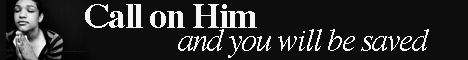Note:  Do not rely on this information. It is very old.

# Figurate Numbers

Figurate Numbers are special series of numbers of interest in the Calculus of Differences. Triangular figurates are based on the series of natural numbers 1, 2, 3, 4, 5, calculated by a set of successive additions. Thus the fourth number of the series is the sum of the numbers 1, 2, 3 and 4; the fifth is the sum of the first five, and so on. Thus starting with 12 3 4 5 6 7 etc., the first order of triangular figurates is 1 3 6 10 15 21 28 etc., the second order is 1 4 10 20 35 56 84 etc., the latter series being obtained from the preceding in precisely the same way. Square figurates start with the series 1 3 5 7 9 11 etc., the difference being 2 between consecutive numbers. Pentagonal figurates have as basis the series whose common difference is 3, and so on for higher series.